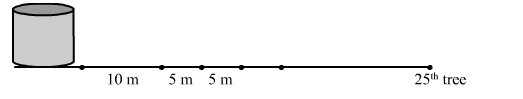# There are 25 trees at equal distances of 5 m in a line with a water tank, the distance of the water tank from the nearest tree being 10 mQuestion:

There are 25 trees at equal distances of 5 m in a line with a water tank, the distance of the water tank from the nearest tree being 10 m. A gardener waters all the trees separately, starting from the water tank and returning back to the water tank after watering each tree to get water for the next. Find the total distance covered by the gardener in order to water all the trees.Solution:

Distance covered by the gardener to water the first tree and return to the water tank = 10 m + 10 m = 20 m

Distance covered by the gardener to water the second tree and return to the water tank = 15 m + 15 m = 30 m

Distance covered by the gardener to water the third tree and return to the water tank = 20 m + 20 m = 40 m and so on.

∴ Total distance covered by the gardener to water all the trees = 20 m + 30 m + 40 m + ... up to 25 terms

This series is an arithmetic series.

Here, a = 20, d = 30 − 20 = 10 and n = 25

Using the formula, $S_{n}=\frac{n}{2}[2 a+(n-1) d]$, we get

$S_{25}=\frac{25}{2}[2 \times 20+(25-1) \times 10]$

$=\frac{25}{2}(40+240)$

$=\frac{25}{2} \times 280$

$=3500$

Hence, the total distance covered by the gardener to water all the trees 3500 m.